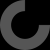# Report as inappropriate

You can calculate the theoretical bearing angle to pitch length (distance per rev.) as [ Pitch = Dia. pi tan(angle) ] or [Angle = arctan(pitch / (dia*pi))] you can calculate the arctangent in the windows scientific calculator by checking the INV box in the upper left corner.

Let's say you want to travel at 200 mm/s with a 600 rpm motor (most should do double or triple that) you will need a 20 mm pitch [mms / (600 / 60)]. Using the angle formula you would need an angle of 38.5 degrees with an 8mm shaft or 32.5 degrees for a 10mm shaft. I believe that the bearings should work up to about 45 degrees if a suitable holder can be made. Of course doubling the rpm would decrease the angle required by half. The possibilities are still there. I will try to make a holder with a 38.5 angle and see. If my math is wrong let me know